## An object of mass 2.95 kg, moving with an initial velocity of 5.02 i hat m/s, collides with and sticks to an object of mass 2.62 kg with an

Question

An object of mass 2.95 kg, moving with an initial velocity of 5.02 i hat m/s, collides with and sticks to an object of mass 2.62 kg with an initial velocity of -3.63 j m/s. Find the final velocity of the composite object.

in progress 0
6 months 2021-08-13T17:42:08+00:00 1 Answers 4 views 0

The final velocity of the composite object is 3.14 m/s.

Explanation:

Given that,

Mass of object 1,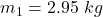Initial speed of object 1,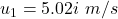Mass of object 2,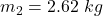Initial speed of object 2,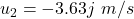The two objects stick together. The momentum of two objects before and after remains constant. Let V is the final velocity of the composite object. using the conservation of linear momentum as :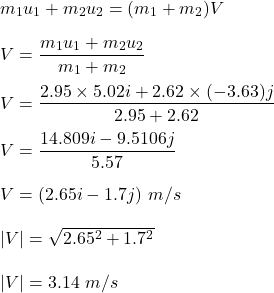So, the magnitude of final velocity of the composite object is 3.14 m/s.